## In the Real World

We here at Shmoop R Us would be remiss if we didn't provide you with some real world information and advice regarding all these sequences and series. This is especially true considering all the different applications they have. Besides, we know how boring the straightforward problems can be sometimes. So bombs away…

If you like applications, and there are many more, don't be afraid to take a look our Best of the Web section.

### Sample Problem

A local radio station is holding a contest to help people get to know the newly elected mayor. The morning DJ is asking listeners to call in and name the new mayor's favorite movie. If the third caller does not name the movie correctly, the \$100 prize money is increased by \$50 for the next day. This \$50 addition continues every morning until a caller answers correctly. If no one answers until the 11th day, how much will the lucky caller win?

Let's be honest, this isn't a monster of a problem to tackle by hand. However, it'll be quicker to use an arithmetic sequence. After all, an arithmetic sequence is created whenever we have the same amount added or subtracted over and over again.

Remember the explicit form for an arithmetic sequence looks like:

{an} = a1 + d(n – 1)

In our situation, the first prize was \$100, so that's a1. The common difference is \$50, which we should put in for d.

{an} = 100 + 50(n – 1)

Since we would like to find the prize money after 11 days, we can just throw 11 in for n and calculate.

a11 = 100 + 50(11 – 1)
a11 = 100 + 50(10)
a11 = 100 + 500
a11 = 600

So the lucky winner will earn a healthy sum of \$600 for calling in and randomly guessing Dumb and Dumber. What can we say? The mayor has great taste.

### Sample Problem

The rival radio station is holding a contest to help people get to know the newly elected mayor as well. They're competitors, what do you expect? Their afternoon DJ is asking listeners to call in and name the new mayor's favorite song. If the fifth caller does not name the song correctly, the \$5 prize money is doubled for the next day. This doubling continues every morning until a caller answers correctly. If no one answers until the 11th day, which station has to pay out more cash to the winner?

A caller on day one would win \$5. A caller on day two would win \$10. Day three: \$20. All of these are barely worth the phone call. But, 11 days…

We could go on and on doubling the prize money by hand until the buttons on our calculators fall off. Or, we could make a rule for a geometric sequence created by this potentially lucrative situation.

This time we're going to need the general form for a geometric sequence:

{an} = a1(r)n 1

The first \$5 prize can be substituted for a1 while the common ratio, r, is 2. This means we have an equation that looks like…

{an} = 5(2)n 1

Since we're after the prize money on the 11th day, 11 once again goes in for n.

a11 = 5(2)11 1
a11 = 5(2)10
a
11 = 5(1024)
a11 = 5120

Ouch! \$5120 later the marketing intern at the rival radio station has resigned for personal reasons. How was anyone supposed to know the mayor was a closet Justin Bieber fan?

### Sample Problem

You've just graduated from a fine institution and as a graduation present received a cool \$1000 cash from a really eccentric, very rich great aunt. Score. The kicker is she made her money via her impressive investment portfolio. Auntie Em has taken it upon herself to invest this \$1000. She has guaranteed you 8% interest over the next 20 years, at which time the money will be yours.

How much will the money be worth when you finally get your hands on it?

Let's start with what we can figure out easily. Our interest here is going to compound annually, so at the end of the first year the gift will be worth…

\$1000 + \$1000(8%) =
\$1000 + \$1000(0.08) =
\$1000 + \$80 =
\$1080

At the end of the second year the gift will be worth…

\$1080 + \$1080(8%) =
\$1080 + \$1080(0.08) =
\$1080 + \$86.40 =
\$1166.40

The third year…

\$1166.40 + \$1166.40(8%) =
\$1166.40 + \$1166.40(0.08) =
\$1166.40 + \$93.31 =
\$1259.71

Things are looking up. Unfortunately, while the gift is meant to teach us patience, we have none at this point. We certainly don't have enough to do this process 20 times.

Luckily, we have a bit of a pattern here. We have gone from a gift worth \$1000 to \$1080, \$1166.40, and then \$1259.71. Dividing any of these values by the previous year's value gives us a common ratio of 1.08. This means that \$1259.71 is really just \$1000(1.08)(1.08)(1.08). We simply need to apply this increase 20 times.

Writing this as a geometric sequence, we have:

an = a1(r)n –1
an
= 1000(1.08)n –1

Notice that we'll have to go to n = 21 for our solution because n = 1 actually gives us our initial value after zero years. This isn't a big deal and simply means the years are just shifted by one in our equation.

a21 = 1000(1.08)21 1
a
21 = 1000(1.08)21 1
a
21 = 1000(1.08)20
a
21 ≈ \$4660.96

Patience is indeed a virtue…if by "a virtue" we mean "worth exactly \$4660.96."

### Sample Problem

A ball is bounced from a height of 10 feet. Each time it bounces, it travels half of its original height. What total distance does the ball travel?

Not counting the initial drop, the ball travels 5 + 5 = 10 total feet on the first bounce: that's 5 feet up and 5 feet back down. It then travels 5 total feet on the second bounce (2.5 + 2.5) and 2.5 feet on the third (1.25 + 1.25). Be careful. Each of these bounces is accounting for the distance the ball travels going up and then down again. So the bounces look like this:

b1 = 10
b2 = 5
b3 = 2.5
b4 = 1.25

Maybe it's just us, but this looks like it could be a geometric series with a first term of 10 and a common ratio of ½. That means we have a series that looks like…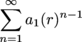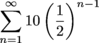All we need to do now is find the sum and then add in the original drop. Since the sum of an infinite geometric series is given by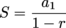we can write the following: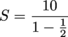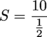S = 20

Of course, we can't forget the original drop: 20 feet + 10 feet = 30 feet. This means the ball travels a total of 30 feet in the air. This is really Shmooptacular, considering the fact that the ball will never really stop bouncing, mathematically speaking. After all, we can always cut a number in half.

• ### I Like Abstract Stuff; Why Should I Care?

In our humble opinion, what you've learned so far is just the tip of the shmathematical iceberg when it comes to sequences and series. There is so much more out there. Some of these ideas will be essential to other courses. Other topics you may never be lucky enough to hear about. Imagine a world where you didn't know what a fractal was. Don't worry; we've got your back.

### Fractals

Take fractals, for instance. If you're into art or graphic design even just a little bit, fractals are a sight for sore eyes. Go ahead, google it. The images are incredible.

What is a fractal? Good question. A fractal is a figure that has what's known as self-similarity. This is a very cool fractal: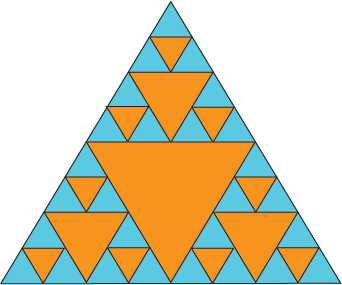This fractal uses equilateral triangles. Notice the pattern. Each time another level of smaller triangles is inserted, more triangles appear. The number of triangles goes from 1 to 3 to 9 to 27. Holy moly. That looks awfully geometric to us. We're thinking a common ratio of 3 with a first term of 1.

Using a geometric sequence is just one way to design a fractal. There are plenty more. However, what's even cooler about fractals is they theoretically go on forever. Think about it like zooming in on an online map. The more you zoom, the more detail you find. Fractals can work in the same way. If we were to zoom into the fractal above, we could find another level with 81 small triangles, then 243, then 729, then 2187, then 6561. You get the idea.

### Calculus II and Then Some

Calculus can sometimes be downright frightening, especially when it's followed by the Roman numeral II. However, after tying up a few loose ends from Calc I, Calc II courses typically jump into sequences and series. This is the real meat and potatoes of most Calc II courses.

This time around, the focus moves mainly to infinite series and proving whether they converge or diverge. Lucky for you, Shmoop has you ready to go with some fancy-pants Calc II vocab. By the way, if you need a refresher, the Glossary might be of assistance.

Where Calc II gets tricky is that there are more series types and tests to learn than just arithmetic and geometric. In fact, once you learn about these other series types, you'll start to create your own series from scratch. You'll even be able to take series and put x's into the rule, like this: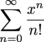Believe it or not, if you start listing the terms of this series, you actually get an infinitely long polynomial.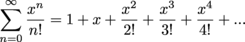What's amazing about this is that, for reasons you'll discover when Calc II finally rolls around, this series is actually a polynomial version of ex. And by a polynomial version, we mean that if you graphed this polynomial with all of its terms, it would be exactly the same as the graph of ex. Identical.

We find this to be fascinating. Especially when we can do the same thing for the graphs of sine and cosine. After all, how do you think your calculator evaluates sin(17) so quickly?